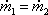This equation is called the continuity equation. It is a statement of the fact that in the case of an incompressible fluid flowing through a pipe that is completely full of the fluid, the mass flow rate at any position of the pipe is the same as the mass flow rate at any other position in the pipe.

Let positions 1 and 2 represent the ends of a segment of the pipe. The continuity equation reflects the fact that the amount of fluid that flows in one end of the pipe segment per second must be equal to the amount of fluid that flows out the other end of the pipe segment per second.